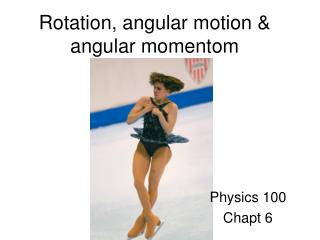Download PresentationRotation, angular motion & angular momentom

Loading in 2 Seconds...

# Rotation, angular motion & angular momentom - PowerPoint PPT Presentation

Rotation, angular motion & angular momentom. Physics 100 Chapt 6. Rotation. Rotation. d 2. d 1. The ants moved different distances: d 1 is less than d 2. Rotation. q. q 2. q 1. Both ants moved the Same angle: q 1 = q 2 (= q ). Angle is a simpler quantity than distanceI am the owner, or an agent authorized to act on behalf of the owner, of the copyrighted work described.
Download Presentation## Rotation, angular motion & angular momentom

An Image/Link below is provided (as is) to download presentation

Download Policy: Content on the Website is provided to you AS IS for your information and personal use and may not be sold / licensed / shared on other websites without getting consent from its author.While downloading, if for some reason you are not able to download a presentation, the publisher may have deleted the file from their server.

- - - - - - - - - - - - - - - - - - - - - - - - - - E N D - - - - - - - - - - - - - - - - - - - - - - - - - -
Presentation Transcript
1. Rotation, angular motion & angular momentom Physics 100 Chapt 6

2. Rotation

3. Rotation d2 d1 The ants moved different distances: d1 is less thand2

4. Rotation q q2 q1 Both ants moved the Same angle: q1 =q2 (=q) Angle is a simpler quantity than distance for describing rotational motion

5. Angular vs “linear” quantities Linear quantity symb. Angular quantity symb. distance d angle q velocity v angular vel. w change in d elapsed time = change in q elapsed time =

6. Angular vs “linear” quantities Linear quantity symb. Angular quantity symb. distance d angle q velocity v angular vel. w acceleration a angular accel. a change in w elapsed time change in v elapsed time = =

7. Angular vs “linear” quantities Linear quantity symb. Angular quantity symb. distance d angle q velocity v angular vel. w acceleration a angular accel. a mass m Moment of Inertia I (= mr2) resistance to change in the state of (linear) motion resistance to change in the state of angular motion moment arm M Moment of inertia = mass x (moment-arm)2 x

8. Moment of inertial M M x I  Mr2 r r r = dist from axis of rotation I=small I=large (same M) easy to turn harder to turn

9. Moment of inertia

10. Angular vs “linear” quantities Linear quantity symb. Angular quantity symb. distance d angle q velocity v angular vel. w acceleration a angular accel. a mass m moment of inertia I Force F (=ma) torque t (=I a) Same force; bigger torque Same force; even bigger torque torque = force x moment-arm

11. Teeter-Totter His weight produces a larger torque F Forces are the same.. but Boy’s moment-arm is larger.. F

12. Angular vs “linear” quantities Linear quantity symb. Angular quantity symb. distance d angle q velocity v angular vel. w acceleration a angular accel. a mass m moment of inertia I torque t (=I a) Force F (=ma) angular mom. L(=I w) momentum p (=mv) Iw = Iw Angular momentum is conserved: L=const

13. Conservation of angular momentum Iw Iw Iw

14. High Diver Iw Iw Iw

15. Angular momentum is a vector Right-hand rule

16. Conservation of angular momentum Girl spins: net vertical component of L still = 0 L has no vertical component No torques possible Around vertical axis vertical component of L= const

17. Turning bicycle These compensate L L

18. Torque is also a vector example: pivot point another right-hand rule F t is out of the screen Thumb in t direction wrist by pivot point F Fingers in F direction

19. Spinning wheel t wheel precesses away from viewer F

20. Angular vs “linear” quantities Linear quantity symb. Angular quantity symb. distance d angle q velocity v angular vel. w acceleration a angular accel. a mass m moment of inertia I torque t (=I a) Force F (=ma) momentum p (=mv) angular mom. L(=I w) kinetic energy ½mv2 rotational k.e. ½I w2 w I V KEtot = ½mV2 + ½Iw2

21. Hoop disk sphere race

22. Hoop disk sphere race I KE = ½mv2 + ½Iw2 KE = ½mv2 + ½Iw2 I KE = ½mv2 + ½Iw2 I

23. Hoop disk sphere race Every sphere beats every disk & every disk beats every hoop